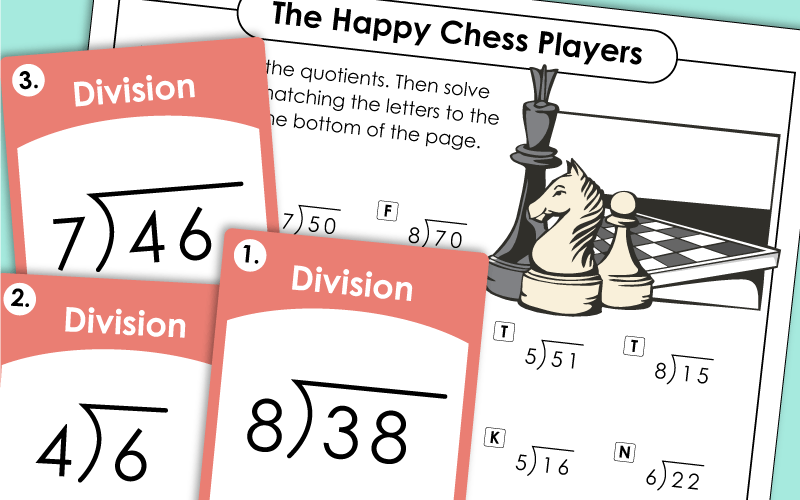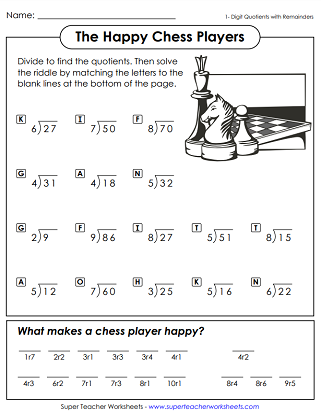# Basic Division with Remainders (2-Digit Dividends)

These printable worksheets can be used to review division (2-digit dividends) with remainders. Quotients have 1 or 2 digits. Download printable PDF worksheets, task cards, and puzzles.## 2-Digit Dividends (Remainders)

Teach the concept of remainders with the help of these pages
Solve basic division problems with remainders. Then use the answers to find the answer to the riddle.
This file has thirty task cards. Use them for learning centers, math games, exit slips, or extra practice.
Solve these division problems; includes 1-digit and 2-digit quotients with remainders. Then color the picture of the boy and his sandcastle.
1 and 2-digit quotients, all problems have remainders; Winter snowmen pictures on the page.
Student rewrite each division problem and solve. Each problem has a 2-digit dividend. The quotients each have 1 or 2 digits. Most problems have remainders.
2 or 3-digit dividends, 2 or 3-digit quotient, problems do not have remainders; Lion picture and word problem.
Division problems with 2-digit dividends and 2-digit quotients.

## Long DivisionWorksheet Generator

Division Worksheet Generator

Create your own long division worksheets! You choose the number of digits in the dividend and the divisor. Also tell if you would like remainders.

## 2-Digit Dividends (Remainders)

Decode the picture symbols and change them into division problems using the key. Then solve.
More Long Division

This index page will help you find long division printables with 2, 3, and 4-digit dividends.

## Sample Worksheet ImagesMy Account
Site Information